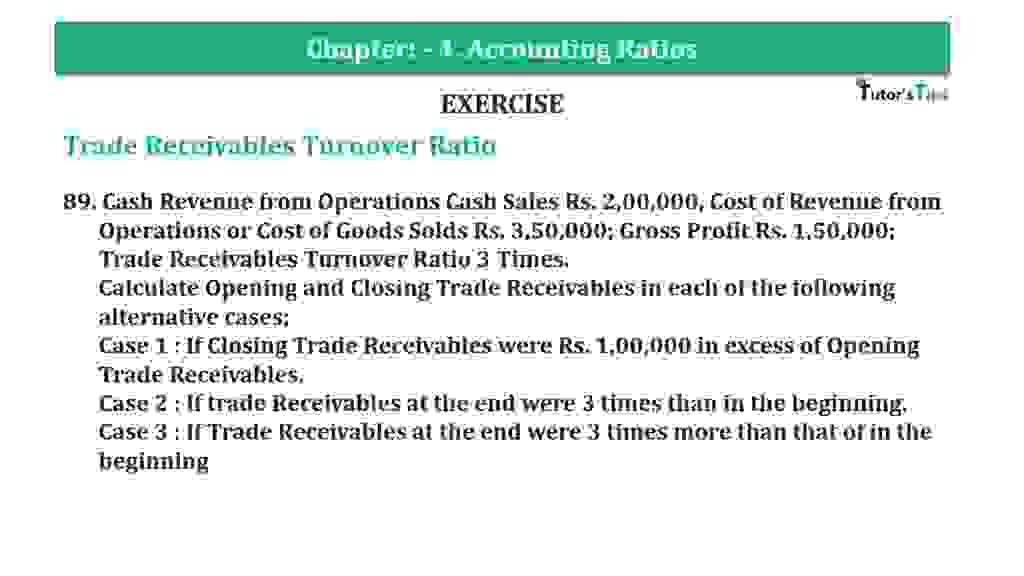# Question 89 Chapter 4 of +2-B – T.S. Grewal 12 ClassQuestion 89 Chapter 4 of +2-B

Trade Receivables Turnover Ratio

89. Cash Revenue from Operations Cash Sales Rs. 2,00,000, Cost of Revenue from Operations or Cost of Goods Sold Rs. 3,50,000; Gross Profit Rs. 1,50,000; Trade Receivables Turnover Ratio 3 Times.
Calculate Opening and Closing Trade Receivables in each of the following alternative cases;
Case 1: If Closing Trade Receivables were Rs. 1,00,000 in excess of Opening Trade Receivables.
Case 2: If trade Receivables at the end were 3 times than in the beginning.
Case 3; If Trade Receivables at the end were 3 times more than that of in the beginning

### The solution of Question 89 Chapter 4 of +2-B: –

 Total Sales = Cost of Goods Sold + Gross Profit = Rs. 3,50,000 + Rs. 1,50,000 = Rs. 5,00,000 Credit Sales = Total Sales − Cash Sales = Rs. 5,00,000 − Rs. 2,00,000 = Rs. 3,00,000

 Trade Receivables Turnover Ratio = Credit Sales Average Trade Receivable
 3 = Rs. 3,00,000 Average Trade Receivable = Rs. 3,00,000 3 Average Trade Receivable = Rs. 1,00,000 Opening Trade Receivables = X Closing Trade Receivables = x + Rs. 1,00,000

 Average Trade Receivable = Opening Trade Receivable + Closing Trade Receivable 2
 Rs. 1,00,000 = X + (x+ Rs. 1,00,000) 2 Rs. 2,00,000 = 2x + Rs. 1,00,000 2x Rs. 2,00,000 – Rs. 1,00,000 2x Rs. 1,00,000 = Rs. 1,00,000 2 X = Rs. 50,000 Opening Trade Receivables = Rs. 50,000 Closing Trade Receivables = Rs. 50,000 + 1,00,000 = Rs. 1,50,000

 Opening Trade Receivables = X Closing Trade Receivables = 3x Average Trade Receivable = Opening Trade Receivable + Closing Trade Receivable 2 Rs. 1,00,000 = X + 3x 2 Rs. 2,00,000 = 4x 4x = Rs. 2,00,000 X = Rs. 2,00,000 4 X = Rs. 50,000 Opening Trade Receivables = Rs. 50,000 Closing Trade Receivables = Rs. 50,000 X 3 = Rs. 1,50,000

 Opening Trade Receivables = X Closing Trade Receivables = X + 3x Average Trade Receivable = Opening Trade Receivable + Closing Trade Receivable 2 Rs. 1,00,000 = x + (x + 3x) 2 Rs. 2,00,000 = 5x 4x = Rs. 2,00,000 X = Rs. 2,00,000 5 X = Rs. 40,000 Opening Trade Receivables = Rs. 40,000 Closing Trade Receivables = Rs. 40,000 X 4 = Rs. 1,60,000

Balance Sheet: Meaning, Format & Examples

Thanks, Please Like and share with your friends

Comment if you have any question.

Also, Check out the solved question of previous Chapters: –

### T.S. Grewal’s Double Entry Book Keeping (Vol. II: Accounting for Companies)T.S. Grewal’s Analysis of Financial Statements